Probability

# Introduction to Recursion: Level 1 Challenges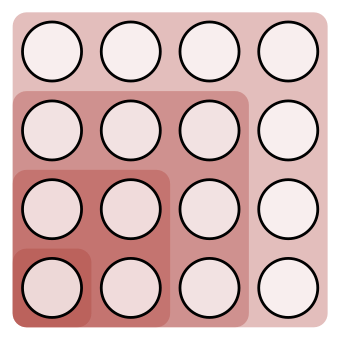Which of the following is demonstrated by the diagram above: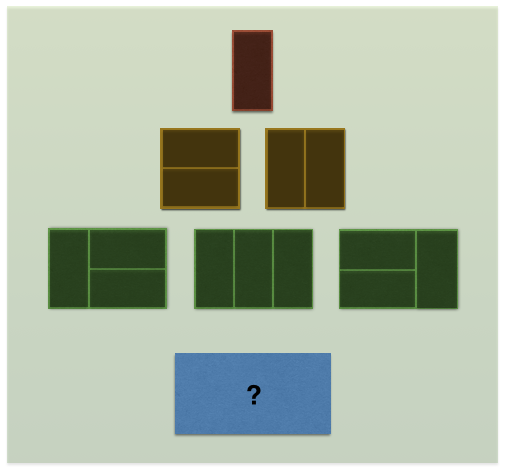Using 1'' x 2'' dominoes, there's one way to tile a 1'' x 2'' area, 2 ways to tile a 2'' x 2'' area, and 3 ways to tile a 3'' x 2'' area.

How many ways are there to tile a 4'' x 2'' area?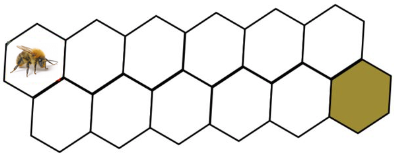Bombus the BumbleBee wants to get to the cell containing honey.

Each time, he can only take a step to a neighboring cell, that moves him to the right.

How many ways does he have of reaching the honey in the last cell?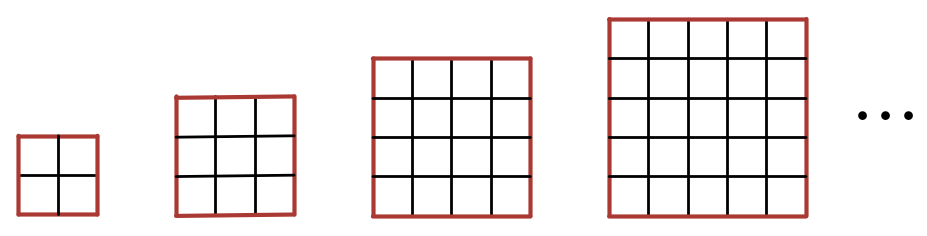Which of the following expresses the correct formula for the number of unit squares with exactly 1 red side in an $n \times n$ square with $n \geq 2$ ?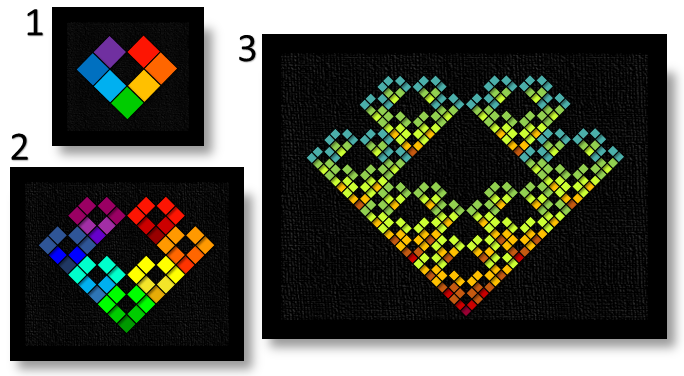What relationship describes the number of mosaic squares in each image compared to the number of squares in the previous image?

×